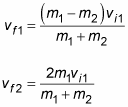# What is the correct collision formula in this scenario?

• StarWarsNerd

#### StarWarsNerd

I am working on a simple space game, and I am having trouble figuring out how to do collisions correctly; things bounce in the wrong direction or just get stuck together. Here are the things that are given:
• Direction of objects A + B in degrees
• Speed of objects A + B in meters per second
• Mass of objects A + B in kilograms
• The material of A + B (any properties of that material)
The collision is in two dimensions, and it is not always going to be head on. What is the formula that I need to simulate the objects colliding?

On a related note: how would I calculate if the objects should spin due to the collision?

Thanks! I had been looking for something like that for days. I'll post back here if I have any other problems.

UPDATE:if I use this and break the final velocities into their x and y components, would I get the desired result?

Last edited: